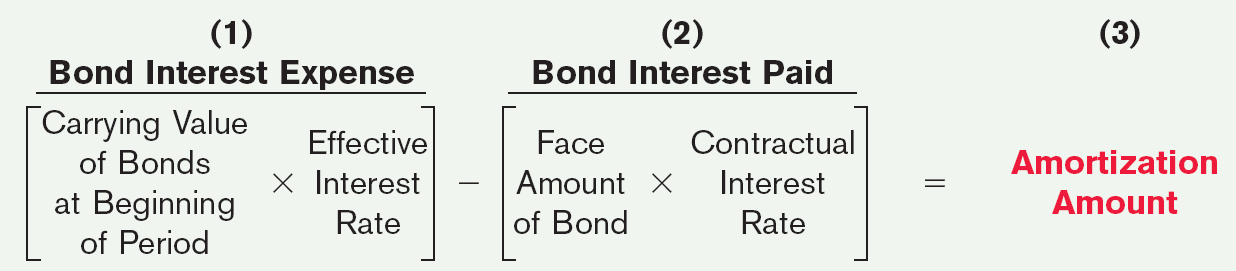# Bond Amortization, Interest Expense, and Interest Payments

Once a bond has been issued and bonds payable liability has been created, the company will pay periodic interest payments to the bond holders for the life of the bond.

The interest payments made to the bondholders are calculated using the coupon rate and the bond’s face value. For example, for a bond with a face value of $1,000 paying a 5% coupon rate, the coupon per year will be$50.

However, the amount of interest expense reported in the income statement will differ from this value depending on whether the bond is issued at par, discount or premium.

 Bond Interest Expense Bond issued at par The interest expense reported on income statement for the period will be equal to the coupon payment. Bond issued at premium Interest expense will be less than the coupon payment.This is because the premium collected (Carrying value – Face value) is amortized over the life of the bond.Total interest expense = Coupon payment – Premium amortizedThe bond liability will be decreased every period equal to the premium amortized. Bond issued a discount Interest expense will be greater than the coupon payment.This is because the discount (Face value – Carrying value) is amortized over the life of the bond.Total interest expense = Coupon payment + Discount amortizedThe bond liability will be increased every period equal to the discount amortized.

There are two methods for amortizing the premium or discount of bonds. They are effective interest method and the straight line method.

The straight line method is just like the straight line method for depreciation. The total premium/discount is divided equally over the life of the bond and these equal amounts are amortized every year.

Under the effective interest method, the interest expense is calculated by multiplying the carrying value of the liability at the beginning of the period by the bond’s yield at issuance.

The amortization amount is then calculated as the difference between the bond interest expense and the bond interest paidThe effective interest method is required under IFRS. Under US GAAP both straight line and effective interest method are allowed but the effective interest method is preferred.

Under US GAAP, cash interest paid is reported as an operating cash flow. Under IFRS, cash interest paid can be reported as operating or financing cash flow.

#### Example

Widgets, Inc. sold $100,000, five-year, 10% bonds on January 1, 2013, for$98,000.  The effective-interest rate is 10.53% and interest is payable on Jan. 1 of each year

We can prepare the bond discount amortization schedule as follows:

 Interest Periods Interest to be paid (10% * 100,000) Interest Expense to be recorded (10.53% * Preceding bond carrying value) Discount Amortization Unamortized discount Bond Carrying Value Issue Date 2000 98000 1 10000 10319 319 1681 98319 2 10000 10353 353 1328 98672 3 10000 10390 390 937 99063 4 10000 10431 431 506 99494 5 10000 10477 506 0 100000 50000 51971 2000

For premium bonds, the amortization works exactly the same way. The only difference is that the interest expense will be lower than the coupon payment by the amount of amortization. The bond carrying value will finally reduce to the bond’s face value.

For a zero-coupon bond, the amortization is exactly like the discount bond. The only difference is that the bond is issued at a deep discount and there are no coupon payments. So, the total interest expense for the year comprises the discount amortization for the year.

### Fair Value Reporting

When a bond is issued, the book value of the bond is the fair value of the bond based on the market interest rate. Depending on where market interest rates stand vis-à-vis bond’s coupon rate, the bond’s carrying value is at premium, par, or discount.

However, if interest rates change, the market value/fair value of bonds will also change. Under both IFRS and US GAAP, the firms have the revocable option to report debt at fair value. Due to a change in market yield, the change in market value of the bond is reported in the income statement as a gain or loss.

Get our Data Science for Finance Bundle for just $29$51.